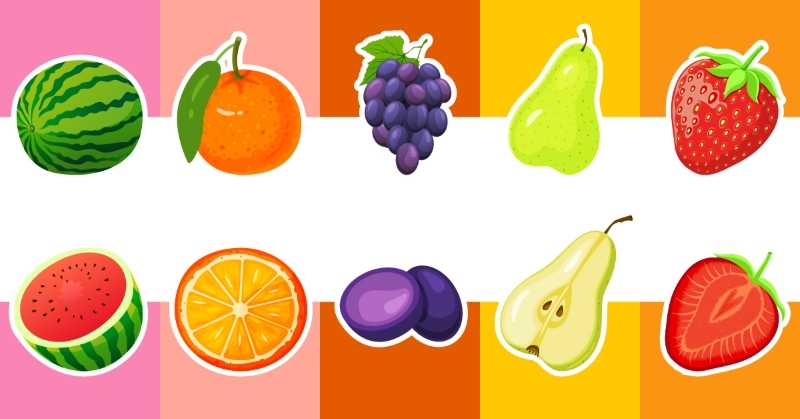Which one is the food that we want?Which one is the animal that we want?Let's do some simple calculations.Can you count these ice creams?Let's count these carrots together!Can you count these trees?Can you find the missing letter?Can you count these ships?Can you tell the first letters of these words of vegetables?How many sandwiches are there?Can you tell the order of these vegetables?Let's count cats together!What are the missing letters?Which one is longer?Which one is the vegetable that we want?Let's count these zebras and horses together!Can you count these corns?Let's count these ducks together!Can you count these radishes and celeries?Can you count these zebras?